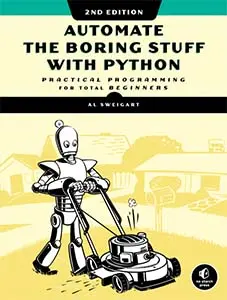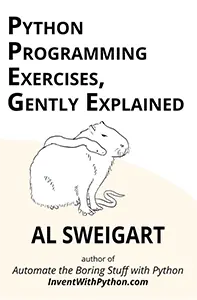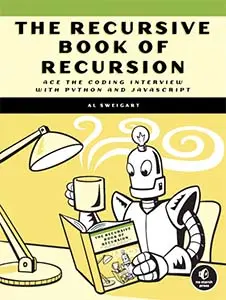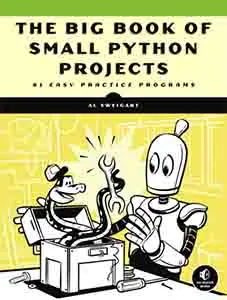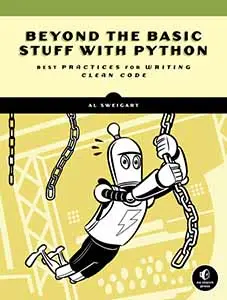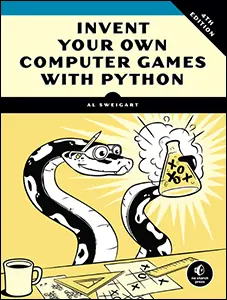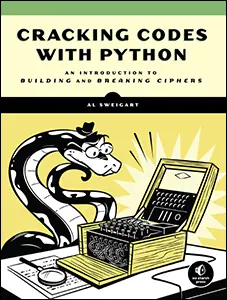# The Invent with Python Blog

Writings from the author of Automate the Boring Stuff.

Mon 20 December 2021

# How to Test Multiple Variables Against a Single Value and a Single Variable Against Multiple Values in Python

Posted by Al Sweigart in python

## TL;DR: Version

To test if a variable is one of many values, use the `in` operator:

```>>> spam = 42
>>> if spam in (3.1415, 'hello', 42, False):
...   print("spam is either 3.1415, 'hello', 42, or False")
...
spam is either 3.1415, 'hello', 42, or False
```

To test if one of multiple variables is one of many values, use a list comprehension and the `any()` function:

```>>> a, b, c = 1, 42, 3
>>> any([x in (100, 3.1415, 'Hello', 42, 'cheese') for x in (a, b, c)])
True
```

## Testing Multiple Variables Against a Single Value And Vice Versa

In Python, if you want to see if a variable is one of many possible values, you could use the `or` operator like this:

```>>> spam = 42
>>> if spam == 3.1415 or spam == 'hello' or spam == 42 or spam == False:
...   print("spam is either 3.1415, 'hello', 42, or False")
...
spam is either 3.1415, 'hello', 42, or False
```

Python has a more concise syntax that uses the `in` operator and the multiple values are packaged in a tuple:

```>>> spam = 42
>>> if spam in (3.1415, 'hello', 42, False):
...   print("spam is either 3.1415, 'hello', 42, or False")
...
spam is either 3.1415, 'hello', 42, or False
```

The `in` operator literally checks if the value in `spam` is in the tuple on the right side of the `in` operator. This code is more readable and in most cases code readability is more important than performance. But using the `in` does perform slightly faster than the series of `or` operators. We can use Python's built-in `timeit` modules to see how many seconds running this code ten million times takes:

```>>> timeit.timeit("spam = 42; spam == 3.1415 or spam == 'hello' or spam == 42 or spam == False", number=10000000)
1.527424699976109

>>> timeit.timeit("spam = 42; spam in (3.1415, 'hello', 42, False)", number=10000000)
0.8834100000094622
```

If you have the opposite case and you have multiple variables you need to check against one value, you can swap the left and right sides of the `in` operator. So instead of using `or` operators like this:

```>>> a, b, c = 3.1415, 'hello', 42
>>> if a == 'hello' or b == 'hello' or c == 'hello':
...   print("One of a, b, or c is equal to 'hello'.")
...
One of a, b, or c is equal to 'hello'.
```

...you can write code using an `in` operator like this:

```>>> if 'hello' in (a, b, c):
...   print("One of a, b, or c is equal to 'hello'.")
...
One of a, b, or c is equal to 'hello'.
```

## Testing Multiple Variables Against Multiple Values

If you have multiple variables and you want to see if any of these variables matches one of several values, you can use a list comprehension and the built-in `any()` function. Let's take a moment to learn about these two concepts first.

The `any()` function takes a list or tuple (or any other iterable value) and returns `True` if any of the values in that list are `True`. If the list only has `False` values or is empty, it returns `False`:

```>>> any([False, False, True, False])
True
>>> any([True, True])
True
>>> any([False, False, False, False, False])
False
>>> any([])
False
```

There is also a built-in `all()` function that returns `True` if the list only contains `True` values, or is an empty list. Otherwise, `all()` returns `False`.

List comprehensions are a short Python syntax for creating list values based on another list or tuple (or any other iterable value).

Normally you would use a `for` loop to create a new list based on another list's values. For example, I'm creating a list called `doubles` which contains the integers in the `numbers` list, but multiplied by `2`:

```>>> numbers = [3, 7, 15]
>>> doubles = []
>>> for number in numbers:
...   doubles.append(number * 2)
...
>>> doubles
[6, 14, 30]
```

A more concise way to write this code in Python is with a list comprehension. Note the similarities between the single-line list comprehension and the multi-line `for` loop code:

```>>> numbers = [3, 7, 15]
>>> doubles = [number * 2 for number in numbers]
>>> doubles
[6, 14, 30]
```

To check if any one of multiple variables contains any one of multiple values, we can use list comprehensions and the `in` operator to create a list of Boolean `True` and `False` values. The list of Booleans created by the list comprehension are based on if the variables' value is in the tuple of values:

```>>> a, b, c = 1, 2, 3
>>> [x in (100, 3.1415, 'Hello', 42, 'cheese') for x in (a, b, c)]
[False, False, False]
>>> b = 42
>>> [x in (100, 3.1415, 'Hello', 42, 'cheese') for x in (a, b, c)]
[False, True, False]
```

We can pass this list of Booleans to the `any()` function and determine if any of the variables matches any of the values:

```>>> a, b, c = 1, 2, 3
>>> any([x in (100, 3.1415, 'Hello', 42, 'cheese') for x in (a, b, c)])
False
>>> b = 42
>>> any([x in (100, 3.1415, 'Hello', 42, 'cheese') for x in (a, b, c)])
True
```

Putting this much code into a single line stretches the limits of code readability, but it is more concise than not using list comprehensions and `any()`. Without them, you'd have to use nested `for` loops and so many lines of code that it's not necessarily more

```>>> a, b, c = 1, 2, 3
>>> match = False
>>> for variable in (a, b, c):
...   for value in (100, 3.1415, 'Hello', 42, 'cheese'):
...     if variable == value:
...       match = True
...
>>> match
False
```

That's a lot of code to do a simple task. The list comprehension and `any()` approach is more concise and readable.